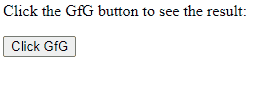GFG App
Open AppBrowser
Continue

# Javascript Error and Exceptional Handling With Examples

An error is an action that is inaccurate or incorrect. There are three types of errors in programming which are discussed below:

• Syntax error
• Logical error
• Runtime error

Syntax error: According to computer science, a syntax error is an error in the syntax of a sequence of characters or tokens that is intended to be written in a particular programming language or it is also a compile-time error if the syntax is not correct then it will give an error message.

Example:

## HTML

 `<``script` `type``=``"text/javascript"``>`   `    ``// An runtime error here` `    ``window.printme();`   ``

As the syntax is not correct of the JavaScript it will affect only the thread that is under this JavaScript and the rest of the code in other threads gets executed as nothing in them depends on the code containing the error.

Logical error: It is the most difficult error to be traced as it is the error on the logical part of the coding or logical error is a bug in a program that causes it to operate incorrectly and terminate abnormally (or crash).

Runtime Error: A runtime error is an error that occurs during the running of the program, also known as the exception. In the example that is given below the syntax is correct, but at runtime, it is trying to call a method that does not exist.

Example:

## Javascript

 ``

As in runtime errors, there are exceptions and these exceptions can be handled with the help of the try-and-catch method

try-catch method

JavaScript uses the try catch and finally to handle the exception and it also uses the throw operator to handle the exception. try have the main code to run and in the catch block if any error is thrown by try block will be caught and the catch block will execute. Finally block will always occur even if an error is thrown

```Note:- We can create our own errors using throw but error thrown can only be
String, Number, Boolean, or an object```

Syntax:

## Javascript

 ``

Example 1:

## HTML

 `` `<``html` `lang``=``"en"``>` `<``head``>`   `    ``<``title``>Document` ``   `<``body``>` `    ``<``p` `id``=``"gfg"``>` `        ``Click the GfG button to see the result:` `    ```   `    ``<``form``>` `        ``<``input` `type``=``"button"` `value``=``"Click GfG"` `onclick``=``"First();"` `/>` `    ``` `    ``<``script` `type``=``"text/javascript"``>` `        ``function First() {` `            ``let a = 123` `            ``let b = 145`   `            ``try {` `                ``document.getElementById('gfg').innerHTML +=` `                    ``'<``br``> Division of ' + a + ' by ' + b + ' = ' + a / b` `            ``}` `            ``catch (e) {` `                ``alert(e.description)` `            ``}` `        ``}` `    ``` `` ``

Output:Example 2:  In this example, use the finally method which will always execute unconditionally after the try/catch.

## HTML

 `` `<``html` `lang``=``"en"``>` `<``head``>`   `    ``<``title``>Document` ``   `<``body``>` `    ``<``script``>` `        ``function First() {` `            ``let a = 123;` `            ``let b = 0;` `            ``try {` `                ``if (b == 0) {` `                    ``throw "Do not divide by zero";` `                ``}` `            ``}` `            ``catch (e) {` `                ``document.getElementById('gfg').innerHTML += ` `                      ``"<``br``>" + e + "<``br``>";` `            ``}` `            ``finally {` `                ``document.getElementById('gfg').innerHTML += ` `                      ``"  Finally block will always execute!";` `            ``}` `        ``}` `    ``` `    ``<``p` `id``=``"gfg"``>Click the GfG button to see the result:` `    ``<``form``>` `        ``<``input` `type``=``"button"` `value``=``"Click GfG"` `onclick``=``"First()"` `/>` `    ``` `` ``

Output:My Personal Notes arrow_drop_up